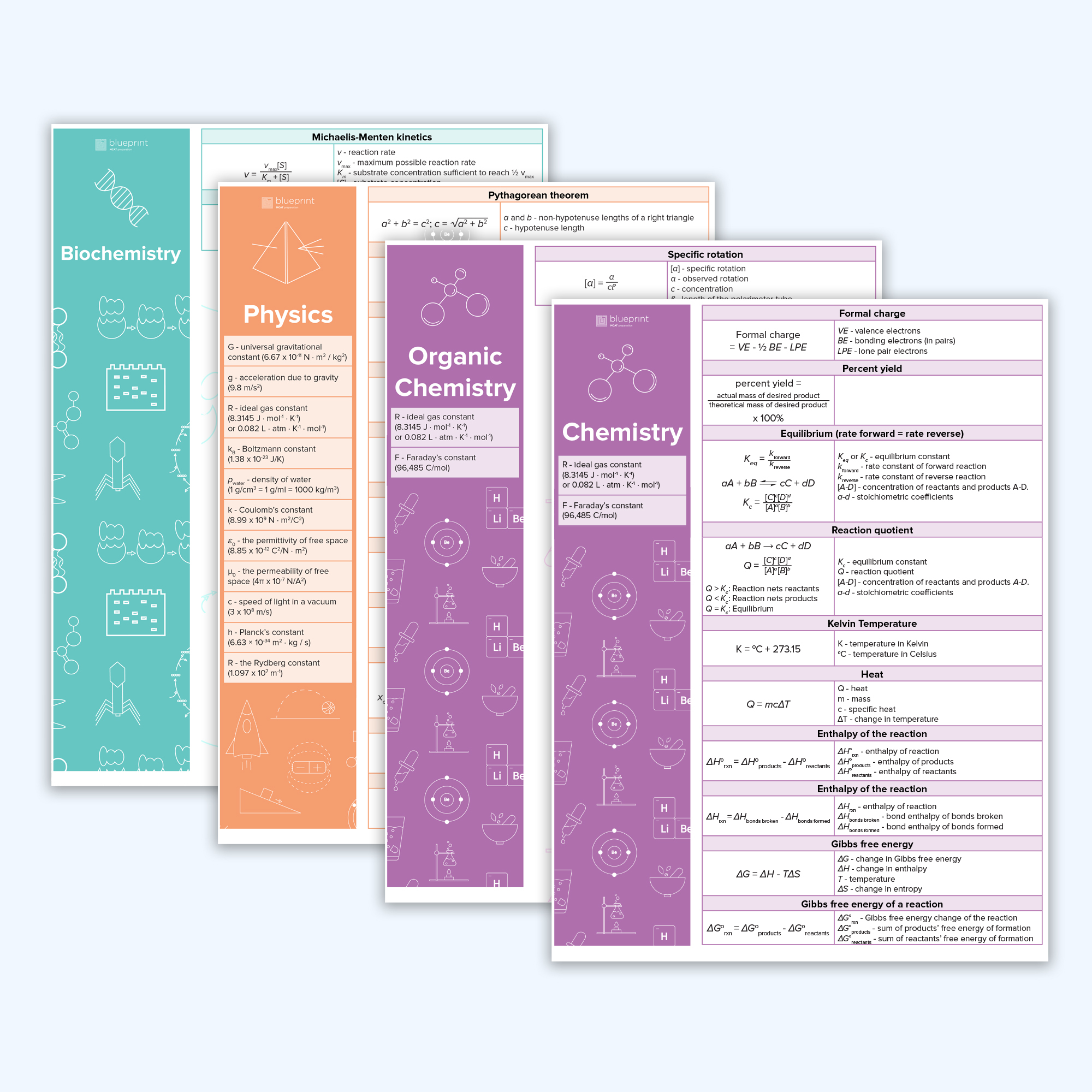FREE MCAT STUDY GUIDE

# Equation SheetsDownload our FREE Equation Sheets! It’s all the physics formulas and chemistry equations you need to know for the Chemical and Physical Foundations of Biological Systems Section of the MCAT. Use these equation cheat sheets to memorize these formulas and equations so you can feel confident and prepared for that section of the exam.

## Equation Sheets You'll Actually Use!

Ah, physics! You know this: What goes up must come down. But hopefully not your MCAT score! But then there’s always chemistry and mixing those different colored liquids together. Fun, right? Turns out, it’s a bit harder than that. And by a bit, we mean a lot!

Whether it’s chemistry or physics that is your most anxiety-inducing subject, (hey, they’re both loaded with math, equations, and formulas!), neither has leeway for mistakes. If you’re going to crush the MCAT, you’re going to have to know all the equations and formulas like you know your parents’ phone number!

Sure you can dive into textbooks to learn them all, but they’re buried between page after page of text. And you’ll need multiple chemistry and physics textbooks to find them all.

Not anymore! Download our FREE Equation Sheets and get all of your chemistry equations AND physics formulas in one place, all in an easy-to-read format!

These Equation Sheets contain every equation formula you’ll need to master Chemical and Physical Foundations of Biological Systems Section of the MCAT, including:

• Equilibrium, Gibbs free energy, reaction rates, pH calculations, and more
• Definitions of variables and terms to make comprehension easy
• Reminders of the different constants you’ll use
• The highest yield physics formulas and content
• The values of physics constants
• Definitions of variables and terms to make comprehension easy
• The complete equation set for kinematics, fluid dynamics, soundwaves, and more!